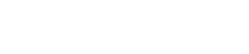Support_Pages

​Products

## Phase vs. Temperature Relationship

COMPARISON OF SOLID PTFE AND MICROPOROUS PTFE SEMI-RIGID CABLESPerformance shown is based on a 25 foot section of .141 diameter microporous cable with an approximate velocity of 81.5%. Actual results will be highly dependant on cable velocity and test procedure used. The factory should be consulted for specific phase temperature performance of a given cable type.

CALCULATE PHASE SHIFT VERSUS TEMPERATURE

To determine a rough estimate of the phase shift (due to temperature) that is contributed to your system by any particular semi-rigid cable assembly or assemblies;

FIRST find the difference in PPM between the two temperatures in question from the above plot. (Other sizes of solid and microporous Teflon cables have plots that are similar to the ones above.)

THEN calculate the phase shift (0) using= .00036 x PPM x L x T x F where:= Predicted phase shift in degrees
PPM = Difference in PPM between the two temperatures in question (from the above plot)
L = Total length of cable that is exposed to the temperature change (expressed in feet)
T = Time delay (ns/ft). This is approximately 1.25 for our microporous PTFE cables and
1.44 for solid PTFE cables
F = Frequency in GHz